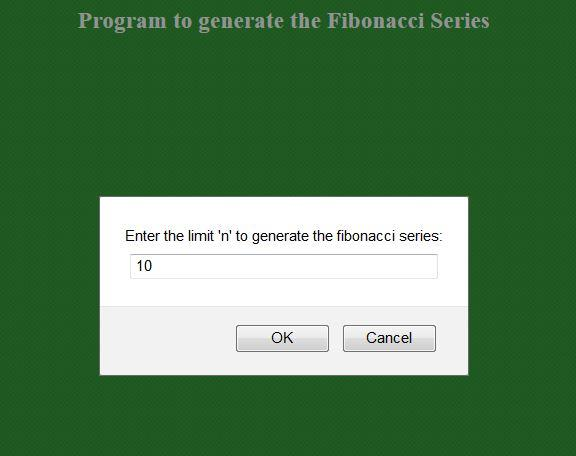How to generate Fibonacci numbers using Javascript

By | April 9, 2017

This program explains how to generate Fibonacci numbers using Javascript. The javascript is embedded in HTML code. You can refer to the input and output images attached.

Input: A number
Output: Fibonacci numbers series for the entered number

//fibonacci.html

<!DOCTYPE HTML>
<?xml version="1.0" encoding="UTF-8"?>
<html>
<title> fibonacci.html </title>
<body style="background-color:green"> <!-- it is advised to not use inline styling -->
<h3 style="text-align:center; color:white"> Program to generate the Fibonacci Series </h3>
<script type="text/javascript">

var limit = prompt("Enter the limit 'n' to generate the fibonacci series:", " ");
var f1=0;
var f2=1;

document.write("The limit entered to generate the fibonacci series is: ",limit, "<br/>");
document.write("The fibonacci series : ");
document.write("",f1," ");
document.write("",f2," ");

var i,f3;
for(i=2; i<limit; i++)
{
f3=f1+f2;
document.write("",f3," ");
f1=f2;
f2=f3;
}

</script>
</body>
</html>

Input:Output:

How to execute this script?

1. Copy the code to a text file and save it with a .html extension.
2. Open this file in a web browser.
3. You will be asked to enter a number and as a result, the corresponding Fibonacci series is displayed for that number.
4. To check for another number, refresh the web page and enter a new number.

Please let me know if you encounter any problems.Author: Ravi Bandakkanavar

A Techie, Blogger, Web Designer, Programmer by passion who aspires to learn new Technologies every day. A founder of Krazytech. It's been 10+ years since I am publishing articles and enjoying every bit of it. I want to share the knowledge and build a great community with people like you.

Suggested articles for you:

3 thoughts on “How to generate Fibonacci numbers using Javascript”

1.ichigo

How can I limit the length to 25 ?

2.Manjunath

This code is not working on my Crome and IE browser… Showing Errors as like below

error on line 8 at column 17: AttValue: ” or ‘ expected
Below is a rendering of the page up to the first error.

Program to generate the Fibonacci Series
and the program is-

fib.html

Program to generate the Fibonacci Series

var limit = prompt(“Enter the limit ‘n’ to generate the fibonacci series:”, ” “);
var f1=0;
var f2=1;
document.write(“The limit entered to generate the fibonacci series is:”,limit, “”);
document.write(“The fibonacci series :”);
document.write(“”,f1,””);
document.write(“”,f2,””);
var i,f3;
for(i=2; i<limit; i++)
{
f3=f1+f2;
document.write(“”,f3,””);
f1=f2;
f2=f3;
}

1.Ravi Bandakkanavar Post author

It works perfect in all the browsers. It cannot be browser dependent.